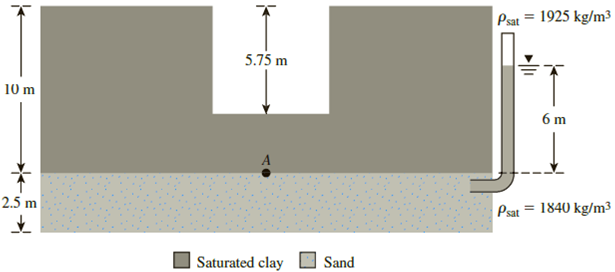Chapter 9, Problem 9.9PPrinciples of Geotechnical Enginee...

9th Edition
Braja M. Das + 1 other
ISBN: 9781305970939

Solutions

Chapter
SectionPrinciples of Geotechnical Enginee...

9th Edition
Braja M. Das + 1 other
ISBN: 9781305970939
Textbook Problem

Refer to Figure 9.28. What would be the maximum permissible depth of cut before heaving would occur?Figure 9.28

To determine

Find the maximum permissible depth (H) of the cut before the occurrence of heaving.

Explanation

Given information:

The thickness h1 of the stiff saturated clay is 10 m.

The depth h2 of the cut is 5.75 m.

The depth (d) of water table is 6.0 m.

The density of the saturated clay ρsat(clay) is 1,925kg/m3.

The density of the sand ρsand is 1,840kg/m3.

Calculation:

Consider the stability of point A in terms of heaving.

Calculate the saturated unit weight γsat(clay) of the clay using the relation.

γsat(clay)=ρsat(clay)γwaterρwater

Here, γwater is the unit weight of the water and ρwater is the density of water.

Take the unit weight of the water as 9.81kN/m3.

Take the density of the water as 1,000kg/m3.

Substitute 1,925kg/m3 for ρsat(clay), 9.81kN/m3 for γwater, and 1,000kg/m3 for ρwater.

γsat(clay)=1,925(9.81)1,000=18.88kN/m3

Calculate the total stress σA at point A using the relation.

σA=(h1H)γsat(clay)

Substitute 10 m for h1 and 18

Still sussing out bartleby?

Check out a sample textbook solution.

See a sample solution

The Solution to Your Study Problems

Bartleby provides explanations to thousands of textbook problems written by our experts, many with advanced degrees!

Get Started

What is the most common type of chart in engineering?

Engineering Fundamentals: An Introduction to Engineering (MindTap Course List)

What is a transfer-type measuring tool?

Precision Machining Technology (MindTap Course List)

What is physical independence?

Database Systems: Design, Implementation, & Management

What is data independence, and why is it lacking in file systems?

Database Systems: Design, Implementation, & Management

List and define the common attributes of tasks within a WBS.

Principles of Information Security (MindTap Course List)

What enterprise resource planning (ERP)? What is supply chain management (SCM)?

Systems Analysis and Design (Shelly Cashman Series) (MindTap Course List)

Define the terms, input and output. List several types of input devices and output devices.

Enhanced Discovering Computers 2017 (Shelly Cashman Series) (MindTap Course List)